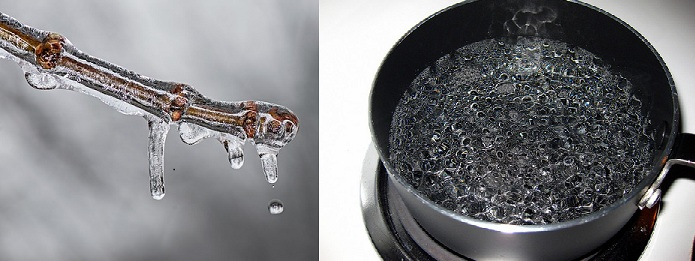# Difference Between Boiling Point and Melting Point

## Main Difference – Boiling Point vs. Melting Point

Melting point and boiling point both describe temperatures at which phase changes occur in substances. The main difference between boiling point and melting point is that the melting point is defined as the temperature at which solid and liquid phases are in equilibrium, whereas the boiling point is the temperature at which the vapour pressure of a liquid is equal to the external pressure.Difference Between Boiling Point and Melting Point – Melting and Boiling

## What is Melting Point

Melting point is defined for a substance as the temperature at which its solid and liquid phases can exist in equilibrium. It is at this temperature that a solid will start melting to  become a liquid. At this temperature, the molecules forming the substance are gaining enough kinetic energy to overcome the intermolecular forces which hold them in fixed positions and they gain the ability to move around. The freezing point describes the temperature at which a liquid can start forming a solid. A substance can be supercooled below its freezing point without it forming a solid, so technically, melting and freezing points are not the same. However, we can assume them to be almost the same for a wide variety of cases.

## What is Boiling Point

Boiling point can be defined for a liquid as the temperature at which its vapour pressure is equal to external pressure. At this temperature, the substance in liquid phase enters the gas phase. If the external pressure is larger, then it takes a higher temperature for the vapour pressure to become equal to the external pressure. Consequently, the boiling point is highly dependent on external pressure. For instance, water can be boiled at much lower temperatures when the external pressure is lower (around 70o C at Mount Everest for example). The  normal boiling point is defined as the temperature at which the vapour pressure of the liquid is equal to 1 atm, i.e. the temperature at which liquid would boil when the external pressure is equal to 1 atm.

## Difference Between Boiling Point and Melting Point

### Definition of Boiling Point and Melting Point

Melting point is the temperature at which solid and liquid phases of a substance are in equilibrium.

Boiling point is the temperature at which its vapour pressure is equal to the external pressure.

### Phase Change

At the melting point, a solid becomes a liquid.

At the boiling point, a liquid becomes a gas.

### Pressure Dependence

Melting point does not change with changes in the external pressure.

Boiling point changes with changes in the external pressure.

###### “Boiling Water” by Scott Akerman (Own work) [CC BY 2.0], via flickr (modified)